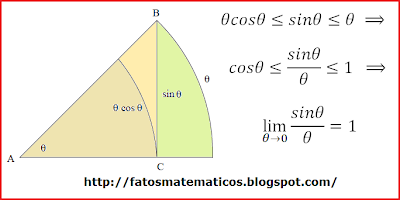# Calculate limits without L'Hospital's Rule

Questions:

(A) Calculate

$$\lim_{x \to \frac{\pi}{2}} \frac{\cot x}{2x - \pi}$$

(B) Calculate

$$\lim_{x ~\to~0^{+}} x^{3}e^{\frac{1}{x}}$$

without using L'Hospital's Rule.

Attempted solution:

(A)

Using the definition of $\cot x$ gives:

$$\lim_{x \to \frac{\pi}{2}} \frac{\cot x}{2x - \pi} = \lim_{x \to \frac{\pi}{2}} \frac{\frac{\cos x}{\sin x}}{2x - \pi} = \lim_{x \to \frac{\pi}{2}} \frac{1}{\sin x} \cdot \frac{\cos x}{2x - \pi}$$

Since the first term will turn out to be 1, I carry on without it and making the substitution $y = x - \frac{\pi}{2}$ as well as the fact that $\cos x = \cos (-x)$:

$$= \lim_{y \to 0} \frac{\cos (y+\frac{\pi}{2})}{2y} = \lim_{y \to 0} \frac{\cos (-y-\frac{\pi}{2})}{2y}$$

Using the subtraction formula for cosine gives:

$$= \lim_{y \to 0} \frac{\cos(-y) \cos \frac{\pi}{2} + \sin(-y) \sin{\frac{\pi}{2}}}{2y} = \lim_{y \to 0} \frac{\sin(-y)}{2y} =$$ $$\lim_{y \to 0} \frac{- \sin(y)}{2y} = -\frac{1}{2}\lim_{y \to 0} \frac{\sin(y)}{y} = -\frac{1}{2} \cdot 1 = -\frac{1}{2}$$

Does this look reasonable?

(B)

I know that the limit does not exist (it "becomes" $\infty$). I figure that $e^{x}$ is continuous function with respect to x and so:

$$\lim_{x ~\to~0^{+}} x^{3}e^{\frac{1}{x}} = \lim_{x ~\to~0^{+}} x^{3} \cdot \lim_{x ~\to~0^{+}} e^{\frac{1}{x}} = 0 \cdot e^{\lim_{x ~\to~0^{+}} \frac{1}{x}}$$

I am not sure where to go on from here. Will it be an argument based on $\frac{1}{x}$ growing faster towards infinity than $x^3$ shrinks towards $0$? Or is there a smart algebraic trick that can be used?

In many cases, the limit appears to give a "zero times infinity" expression, but after figuring out the secret steps you can change it to something that you can calculate the limit from. How do you know when to stop trying and declare that the limit does not exist?

Your first calculation looks good as far as I can tell.

You cannot write $$\lim_{x\to0^+}x^3e^{1/x}=\lim_{x\to0^+}x^3\cdot\lim_{x\to0^+}e^{1/x}$$ because the limit of a product should only be said to equal the product of the limits when all three limits exist. In general, one or both limits on the right might not exist and yet the limit on the left could still exist. It might help to let $z=1/x$, and note that $$\lim_{x\to0^+}x^3e^{1/x}=\lim_{z\to\infty}\frac{e^z}{z^3}$$ Then can you show that for large $z$, $e^z>cz^4$? One method is to

• show that $e^x>1$ for large enough $x$
• show that for some $x_0$, $e^{x_0}>x_0$, and then since we have already established that $e^x$ has a larger derivative than $x$, it follows that for large enough $x$, $e^x>x$
• repeat to show $e^x>\frac{1}{2}x^2$
• repeat to show $e^x>\frac{1}{6}x^3$
• repeat to show $e^x>\frac{1}{24}x^4$

So $$\lim_{x\to0^+}x^3e^{1/x}=\lim_{z\to\infty}\frac{e^z}{z^3}>\lim_{z\to\infty}\frac{cz^4}{z^3}=\infty$$

First limit: your method is reasonable, but too lengthy. I'do the substitution $x-\pi/2=t$ so I have to compute $$\lim_{t\to0}\frac{\cot(t+\pi/2)}{2t}=\lim_{t\to0}\frac{-\tan t}{2t} =\lim_{t\to0}\frac{-1}{2\cos t}\frac{\sin t}{t}$$

Second limit: do the substitution $x=1/t$, so you have to compute $$\lim_{t\to\infty}\frac{e^t}{t^3}= \lim_{t\to\infty}\frac{1}{27}\left(\frac{e^{t/3}}{t/3}\right)^{\!3}$$ so you are reduced to the well known limit $$\lim_{u\to\infty}\frac{e^u}{u}$$ By definition, $$e^u=\sum_{n\ge0}\frac{u^n}{n!}$$ so $e^u>u^2/2$ for $u>0$; thus, for $u>0$, $$\frac{e^u}{u}>\frac{u^2/2}{u}=\frac{u}{2}$$ and finally $$\lim_{u\to\infty}\frac{e^u}{u}=\infty$$

For A. Consider $$\begin{equation*} \frac{1}{\sin x}\frac{\cos x}{2x-\pi }=\frac{1}{2\sin x}\frac{\cos x-\cos \frac{\pi }{2}}{x-\frac{\pi }{2}} \end{equation*}$$ Can you take it from here?

For B: With Asymptotic analysis:

Set $u=\dfrac1x$. We have: $$\lim_{x \to 0^{+}} x^{3}e^{\frac{1}{x}} =\lim_{u\to+\infty}\frac{\mathrm e^u}{u^3}=+\infty$$ since any monomial $u^ k=_{+\infty}o(\mathrm e^u)$

A To prove $\displaystyle\lim_{x \to \infty} \frac{\sin x}{x} = 1$ just look at the wedge of a circle and notice the the arc and the height are about the same. With an appropriate change of angles you can getI think in your cases it might be useful that $\displaystyle \lim_{x \to 0} \frac{\tan x }{x} =1$